# Directional Drilling Calculation Example

I got the question from my friend, Nien, asking me about directional drilling calculation and the question is shown below:

Wellplanner is planning to design a deep kick-off well with a build section to by pass a salt dome section. He intends to achieve a horizontal departure of 1500 ft at the with the TVD at the target point to be 10,000 ft. Calculate the measure depth and build up rate.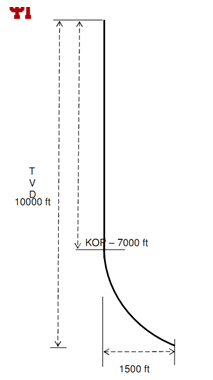Solution:
First thing, I would like to draw a trigonometry diagram like this.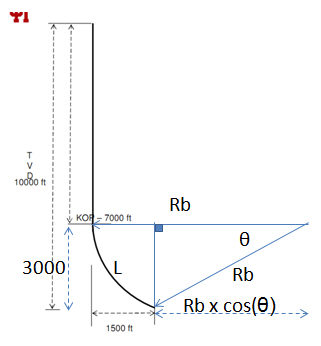Please keep in mind that this diagram I draw with the Power Point so it will not show good proportion but it can give you good idea on how to solve this problem.

The formula for radius build (Rb) and length of hole (L) are listed below respectively;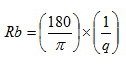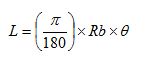Where;
Rb = radius of build in ft
q = build rate degree/ft
θ = angle build in radius

Data given:

Total TVD = 10,000 ft

TVD in build section = 10,000 – 7,000 = 3,000 ft

The horizontal departure = 1500 ft

From the diagram,

Rb = 1500 + Rb x cos (θ)

1500 = Rb x (1- cos (θ)) — >Equation 1

Use the trigonometric relationship

3000 = Rb x sin (θ) — >Equation 2

Equation 2 ÷ Equation 1 so you will get like this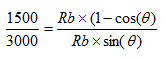— >Equation 3

Square the Equation 3 so you will get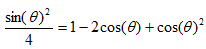— >Equation 4

Arrange the Equation 4 and you will get like this: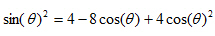— >Equation 5

According to the trigonometry relationship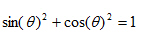Therefore you can change the Equation 5 like this: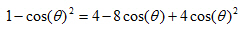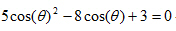— >Equation 6

Use the polynomial relationship to arrange the equation as shown below: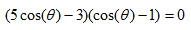You will have two following answers:

cos (θ) = 3/5 and cos (θ) = 1

Then you need to check which answer is correct.

cos (θ) = 1 is unreal because θ = 0 degree.

Therefore, cos (θ) = 3/5 is the right answer for this case.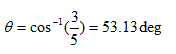From the Equation 1, you will be able to get Rb via the Equation 1

1500 = Rb x (1- cos (θ)) — >Equation 1

1500 = Rb x (1- cos (53.13))

1500 = Rb x (1 – 3/5)

Rb = 3750 ft

You can determine the length of build section via the equation below;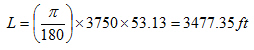Total MD from the rig to end of the build section = 7000 + 3477.35 = 10,477.35 ft

Determine build rate via this following equation: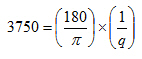q = 0.01528 degree/ft

We can answer in degree/100 ft by multiplied 100 therefore you will get the answer like this.

q = 1.53 degree/100 ft

Conclusion of this question:

The measure depth of the well = 10,477.35 ft

The build-up rate = q = 1.53 degree/100 ft

References

Inglis, T.A. (2010) Directional drilling. Dordrecht: Springer-Verlag New York.

Mitchell, R.F., Miska, S.Z. and Aadnoy, B.S. (2012) Fundamentals of drilling engineering. Richardson, TX: Society of Petroleum Engineers.

Short, J.J.A. (1993) Introduction to directional and horizontal drilling. Tulsa, OK: PennWell Books.

Technical, T., Astier, B., Baron, G., Boe, J.-C., Peuvedic, J.L.P. and French Oil & Gas Industry Association (1990) Directional drilling and deviation control technology. Paris: Editions

Share the joyWorking in the oil field and loving to share knowledge.

### 11 Responses to Directional Drilling Calculation Example

1.Danny Retnadi says:

Very good web to get drilling practices.

2.Kingsley Ekeadah says:

Drilling made easy for young Engineers

3.samy says:

Dear Sir

ton mile calculation formula for running liner casing is the same formula for running casing to surface. please advise

Regards

•DrillingFormulas.Com says:

The formula is the same but the weight of casing is not the same therefore ton-mile for running liner is lower.

4.aras says:

I can not understand the equation:
5cosθ^(2 )- 8cosθ +3 = 0

Can someone explain to me the equation?
tnx

5.Edgar Nova says:

How can I detect a drilling break and a formation change when I am sliding the drill pipe in open hole?

Thank you

•DrillingFormulas.Com says:

ROP change is still a good indicator of drilling break even though you are sliding.

6.Alireza says:

Dear sir
when we are sliding , what can we do when the string is hanging and don’t allow to slide ?

•DrillingFormulas.Com says:

Then try again. Hanging off makes us very difficult to drill. There are several contributing factors affecting these as BHA compoment, formation, hydruluics, drilling parameters, etc.You need to pick up and work few times. Please keep trying.

7.omar hassan says: